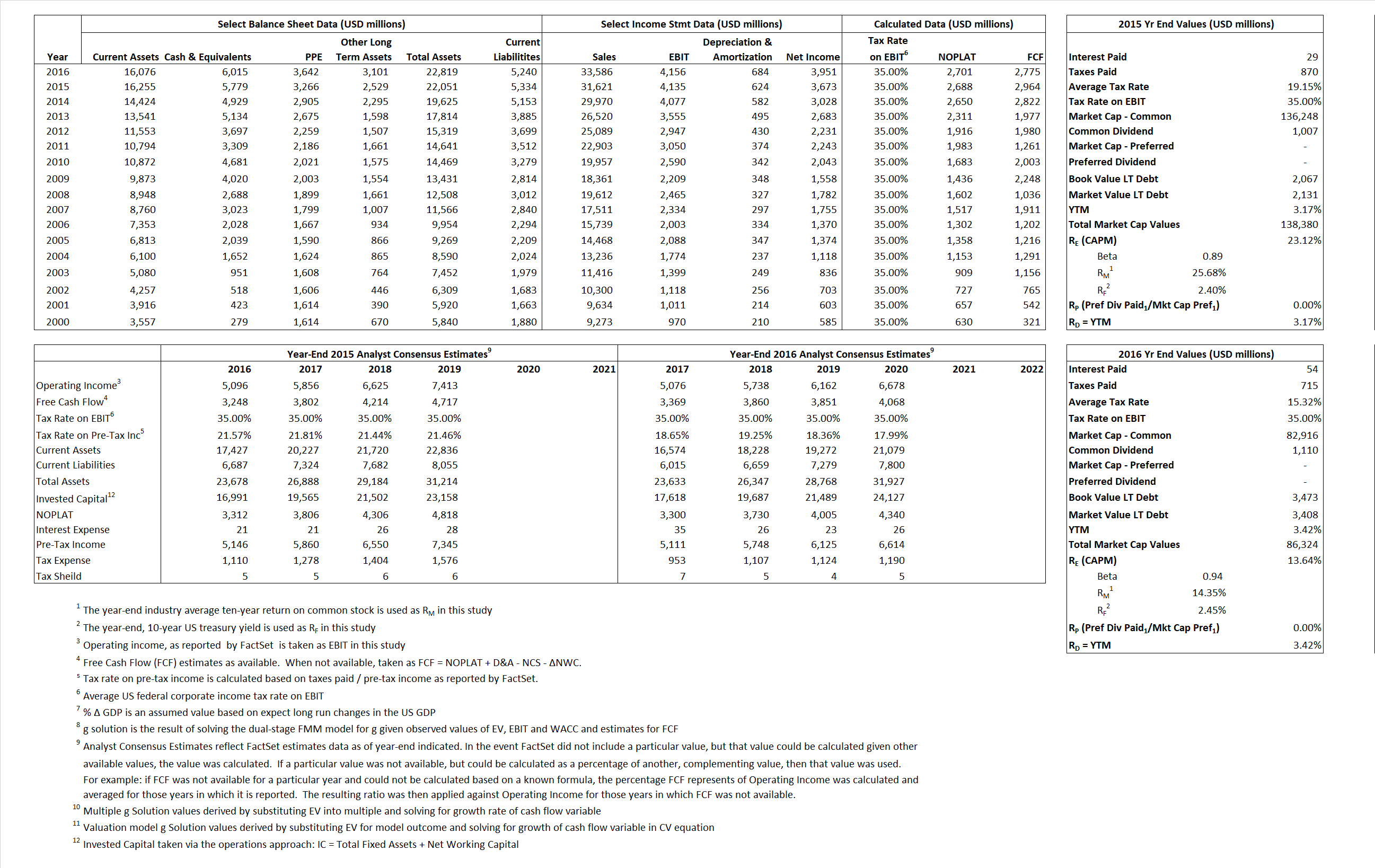# Nike

## Analyst Listing

The following analysts provide coverage for the subject firm as of May 2016:

 Broker Analyst Analyst Email DA Davidson Andrew Burns aburns@dadco.com Canaccord Genuity Camilo Lyon clyon@canaccordgenuity.com Credit Suisse Christian Buss christian.buss@credit-suisse.com Susquehanna Financial Group Christopher Svezia christopher.svezia@sig.com BB&T Capital Markets Corinna L. Freedman cfreedman@bbandtcm.com Jefferies Edward Plank eplank@jefferies.com Brean Capital, LLC Eric B. Tracy etracy@breancapital.com Piper Jaffray Erinn E. Murphy erinn.e.murphy@pjc.com Hilliard Lyons Jeffrey S. Thomison jthomison@hilliard.com Stifel Nicolaus Jim Duffy jduffy@stifel.com Cowen & Company John Kernan john.kernan@cowen.com Telsey Advisory Group Kelly Chen kchen@telseygroup.com B Riley & Co Mitch Kummetz mkummetz@brileyco.com Evercore ISI Omar Saad omar.saad@evercoreisi.com Nomura Research Robert S. Drbul robert.drbul@nomura.com CRT Capital Group Sam Poser sposer@sterneageecrt.com Buckingham Research Scott Krasik skrasik@buckresearch.com FBR Capital Markets & Co Susan Anderson sanderson@fbr.com Wells Fargo Securities Tom Nikic tom.nikic@wellsfargo.com

## Primary Input Data## Derived Input Data

### Equational Form

Net Operating Profit Less Adjusted Taxes NOPLAT  2,688 2,701$NOPLAT\, =\, EBIT\, x\, (1 \,-\, Avg \,\,Tax\,\, Rate\,\, on\,\, EBIT)$
Free Cash Flow FCF 2,964 2,775$FCF\,=NOPLAT\,+\,Non-Cash\,Expenses-\Delta NWC\,-\,NCS$
Tax Shield TS 6 8$TS\,=\,Interest\,\,Paid\,\,x\,\, Avg \,\,Tax\,\,Rate\,\, on\,\, Pre-Tax\,\, Income$
Invested Capital IC 16,717 17,578$IC\,=\,Fixed\,\,Operating\,\,Assets\,\,+\,\,Net\,\, Working\,\, Capital$
Return on Invested Capital ROIC 16.08% 15.37%$ROIC\,=\,\frac { NOPLAT }{ IC }$
Net Investment NetInv 2,869 1,545$NetInv\,=\,{ {IC}_{1}}-{{IC}_{0}}+Depreciation$
Investment Rate IR  106.75%  57.20%$IR\,=\,\frac {NetInv}{NOPLAT}$
Weighted Average Cost of Capital
WACCMarket  22.80% 13.21%$WACC\,=\,\frac { E }{ V } { R }_{ E }\,+\,\frac { P }{ V } { R }_{ P }\,+\,\frac { D }{ V } { R }_{ D }\left( 1- Avg\,\, Tax\,\,Rate\,\,on\,\,Pre-Tax\,\,Income \right)$
WACCBook    8.52%  8.86%
Enterprise value
EVMarket 132,600  80,309$EV\,=\,Market\,\,Cap\,\,Equity\,+\,\,Long\,\,Term\,\,Debt\,-\,Cash$
EVBook  132,300   80,374
Long-Run Growth
g = IR x ROIC
17.16%   8.79% Long-run growth rates of the income variable are used in the Continuing Value portion of the valuation models.
g = %$\Delta$ GDP    2.50%   2.50%
Margin from Operations M   13.08%  12.37%$M\,\,=\,\,\frac{EBIT}{SALES}$
Depreciation/Amortization Rate D   13.11%  14.13%$D\,\,=\,\,\frac{D+A}{EBITDA}$

## Valuation Multiple Outcomes

The outcomes presented in this study are the result of original input data, derived data, and synthesized inputs.

### model g solution

12/31/2015 12/31/2016 12/31/2015 12/31/2016 12/31/2015 12/31/2016

EV/SALES$\frac {EV}{Sales} \,= \,\frac{ROIC\, -\, g}{ROIC\,(WACC\,-\,g)}\,(1\,-\,T)\,(M)$

4.19  2.39  23.77%  12.61%  23.23%  12.88%

EV/EBITDA$\frac {EV}{EBITDA} \,= \,\frac{ROIC\, -\, g}{ROIC\,(WACC\,-\,g)}\,(1\,-\,T)\,(1\,-\,D)$

27.86 16.59 23.77% 12.61% 23.23% 12.88%

EV/NOPLAT$\frac {EV}{NOPLAT} \,= \,\frac{ROIC\, -\, g}{ROIC\,(WACC\,-\,g)}$

49.33 29.73 23.77% 12.61% 23.23% 12.88%

EV/FCFOPS$\frac {EV}{FCF_{OPS}} \,= \,\frac{ROIC\, -\, g}{ROIC\,(WACC\,-\,g)}\,(1\,-\,T)$

44.73 28.94 23.77% 12.61% 23.23% 12.88%

EV/EBIT$\frac {EV}{EBIT} \,= \,\frac{ROIC\, -\, g}{ROIC\,(WACC\,-\,g)}\,(1\,-\,T)$

32.07 19.33 23.77% 12.61% 23.23%  12.88%

EV/IC$\frac {EV}{IC} \,= \,\frac{ROIC\, -\, g}{WACC\,-\,g}$

7.93 4.57 23.77% 12.61% 23.23% 12.88%